# Image Resizing Methods

For use with image resizing functions like GfxResizeImageBlobWithMethod.

The following properties can be set in the resizing method:

• method: how the image should be resized
• setwidth, setheight: the new image dimensions, may not be the actual resulting image dimensions. when a method doesn't require either of these, you can set it to 0 or leave the cell out of the method. (ie [ method := "scale", setwidth := 640, setheight := 0 ] and [ method := "scale", setwidth := 640 ] are equivalent)
• format: output image MIME type, one of "image/jpeg", "image/gif" or "image/png". If left empty, the output image will have the same type as the input image if possible; "image/x-bmp" is converted to "image/png" and "image/tiff" is converted to "image/jpeg".
• bgcolor: the background color to apply when working with (semi-)transparent images or the 'canvas' resizing functions
• quality: if the output image has the "image/jpeg" type, the JPEG quality to use (a value between 0-100)
• fixorientation: try to fysically rotate the image according to the image rotation stored within the image (defaults to true)
• noforce: return the input image if the chosen method and parameters didn't actually change the image (defaults to true)
• grayscale: discard color information in the image (30% red, 59% green, 11% blue)

The method cell is always required, other cells may be required depending on the method.

The image cache will not touch images that already have the correct dimensions and rotation unless the noforce parameter is set. This is a route that can be used to take exact control of the quality and to permit the use of animated GIFs - if the end user does the work to properly resize the image, WebHare won't touch it.

## Image methods

To illustrate the different methods, the following reference image is used: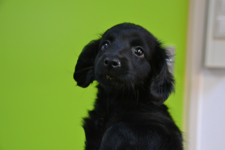(225x150)

### none

The image is left untouched and returned as-is.

## fit

If either the width of the image is larger than setwidth or the height is larger than setheight, the image is scaled down proportionally to fit within setwidth and setheight. If only one of setwidth or setheight is given, only the given direction is checked. This only make the image smaller if it's too big.

[ method := "fit", setwidth := 100, setheight := 100 ]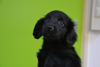(100x67)

[ method := "fit", setwidth := 300, setheight := 300 ](225x150)

[ method := "fit", setwidth := 100 ](100x67)

[ method := "fit", setheight := 100 ]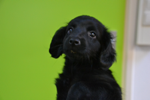(150x100)

[ method := "fit", setwidth := 300 ](225x150)

[ method := "fit", setheight := 300 ](225x150)

## scale

The same as fit, but if the image is also scaled up proportionally if it's smaller than the given setwidth or setheight. Equivalent to CSS: "background-size: contain;".

[ method := "scale", setwidth := 100, setheight := 100 ](100x67)

[ method := "scale", setwidth := 300, setheight := 300 ]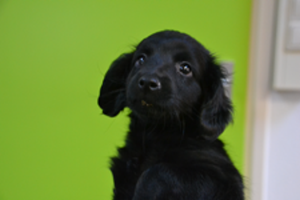(300x200)

[ method := "scale", setwidth := 100 ](100x67)

[ method := "scale", setheight := 100 ](150x100)

[ method := "scale", setwidth := 300 ](300x200)

[ method := "scale", setheight := 300 ]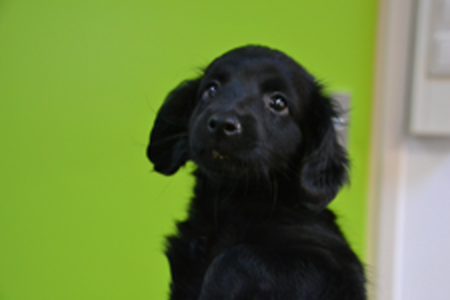(450x300)

## crop

The image is cropped to the given dimensions without being resized. Both setwidth and setheight must be specified.

[ method := "crop", setwidth := 100, setheight := 100 ](100x100)

[ method := "crop", setwidth := 300, setheight := 300 ](225x150)

[ method := "crop", setwidth := 100, setheight := 300 ](100x150)

## fitcanvas, scalecanvas, cropcanvas

The same as fitscale and crop but if both setwidth and setheight are given, the resulting image will always be setwidth by setheight pixels. Any portion of the resulting canvas not covered by the orignal (scaled) image is filled with bgcolor.

[ method := "fitcanvas", setwidth := 100, setheight := 100, bgcolor := "red" ]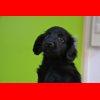(100x100)

[ method := "fitcanvas", setwidth := 300, setheight := 300, bgcolor := "red" ]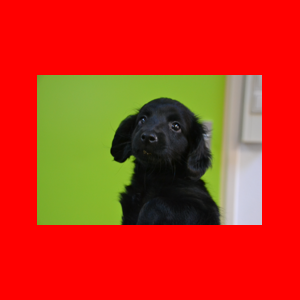(300x300)

[ method := "scalecanvas", setwidth := 100, setheight := 100, bgcolor := "red" ](100x100)

[ method := "scalecanvas", setwidth := 300, setheight := 300, bgcolor := "red" ]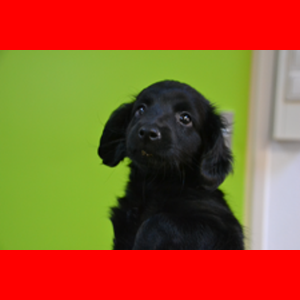(300x300)

[ method := "cropcanvas", setwidth := 300, setheight := 300, bgcolor := "red" ](300x300)

[ method := "cropcanvas", setwidth := 100, setheight := 300, bgcolor := "red" ](100x300)

## fill

The image is scaled proportionally until it covers the setwidth by setheight area. If only one of setwidth and setheight is given, it behaves like the scale method, otherwise equivalent to CSS: "background-size: cover;".

[ method := "fill", setwidth := 100, setheight := 100 ]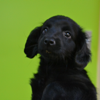(100x100)

[ method := "fill", setwidth := 300, setheight := 300 ]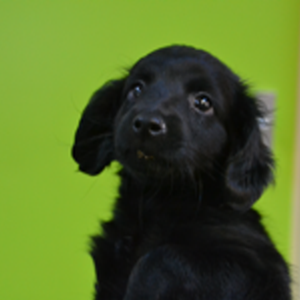(300x300)

## stretch

The image is resized to setwidth by setheight pixels, regardless of its proportions. If only one of setwidth and setheight is given, it behaves like the scale method.

[ method := "stretch", setwidth := 100, setheight := 100 ](100x100)

[ method := "stretch", setwidth := 300, setheight := 300 ](300x300)

## stretch-x, stretch-y

stretch-x scales the image to the width and then constrains the height, resizing if necessary, while stretch-y scales the height and then constrains the width.

[ method := "stretch-x", setwidth := 100, setheight := 100 ](100x67)

[ method := "stretch-x", setwidth := 300, setheight := 300 ](300x200)

[ method := "stretch-y", setwidth := 100, setheight := 100 ](100x100)

[ method := "stretch-y", setwidth := 300, setheight := 300 ](300x300)